# Matrices solutions, Inter maths 1a chapter 3 solutions

Mathematics intermediate first year 1a matrices solutions for some problems for examination purpose.

Study the text book lesson Matrices very well.

Practice the example problems and solutions given in the textbook.

Observe the solutions and try them in your own method.

You can also see

Inter Maths 1A textbook solutions

Inter Maths 1B textbook solutions

Inter Maths IIA textbook solutions

Inter Maths IIB textbook solutions

Matrices

Exercise 3(a)

Exercise 3(c)

Exercise 3(d)

Exercise 3e)

Exercise 3(f)

Exercise 3(g)

Exercise 3(h)

Exercise 3(i)

M

## Matrices inter 1a solutions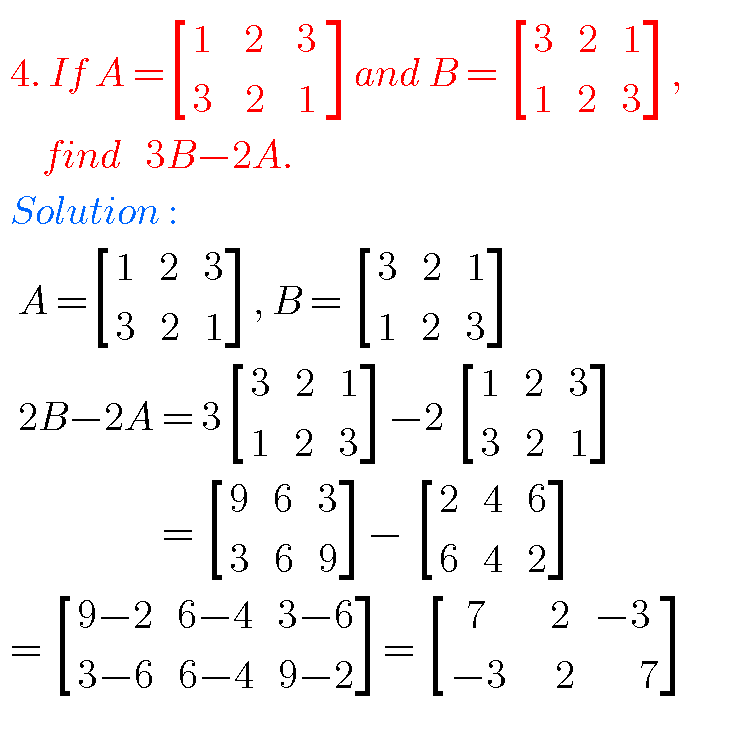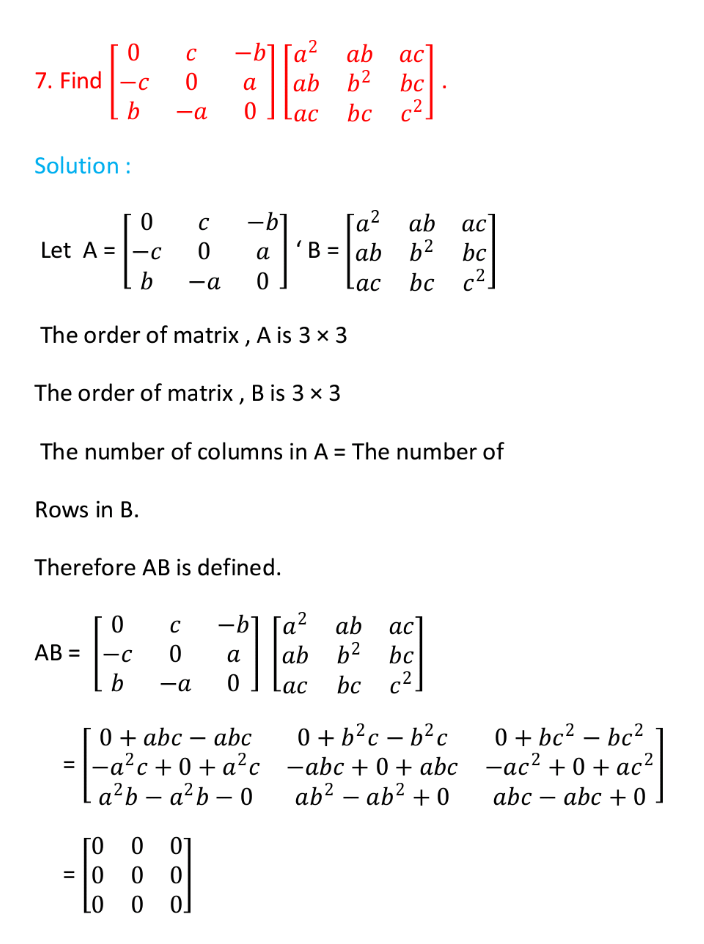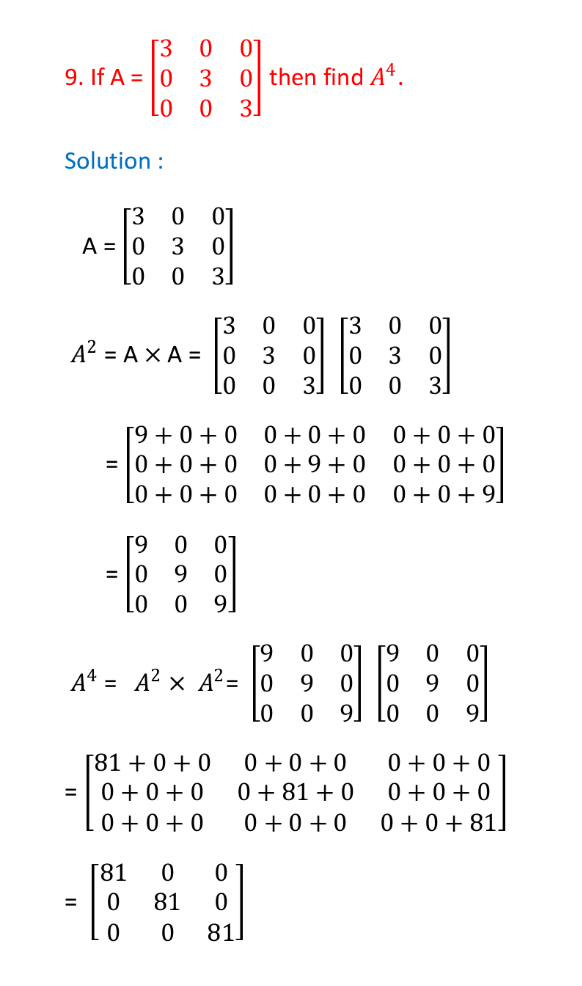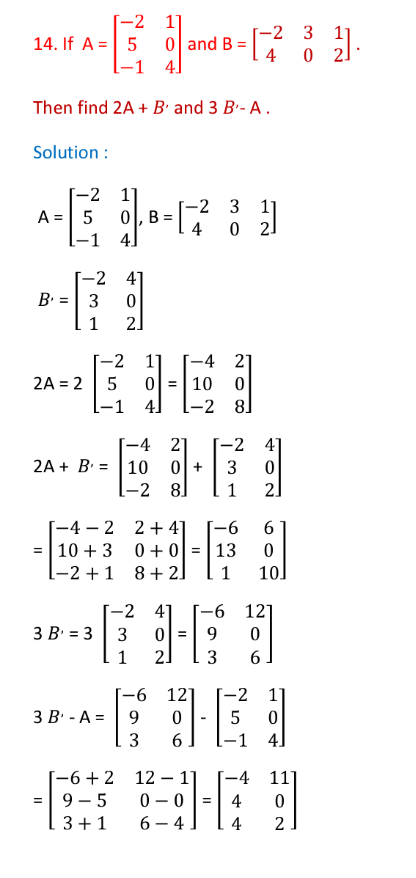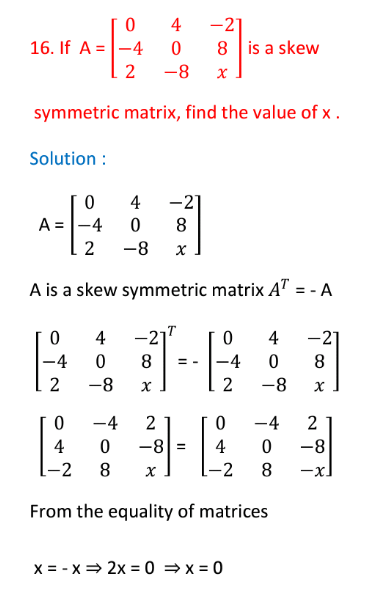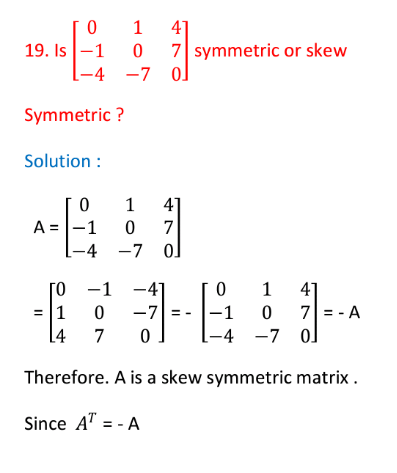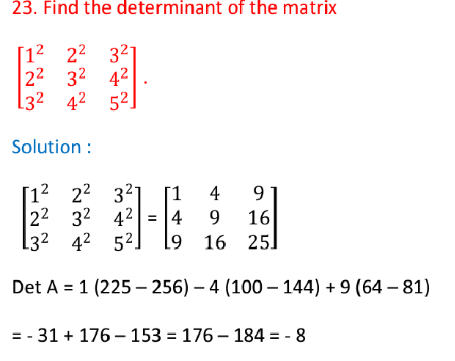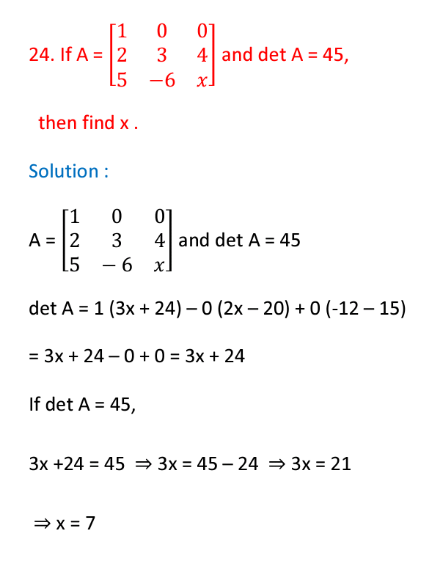### Intermediate matrices solutions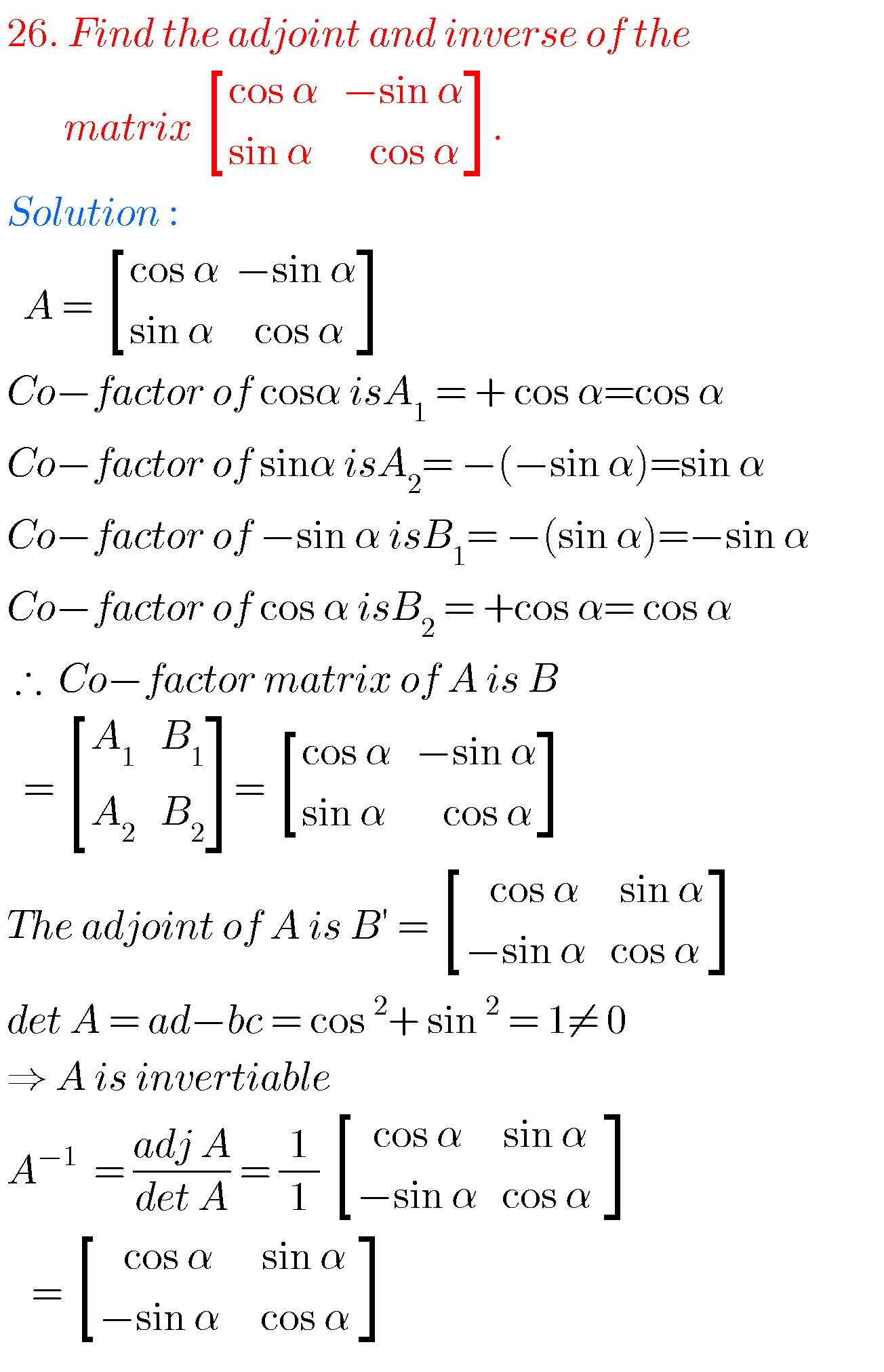## Matrix solutions inter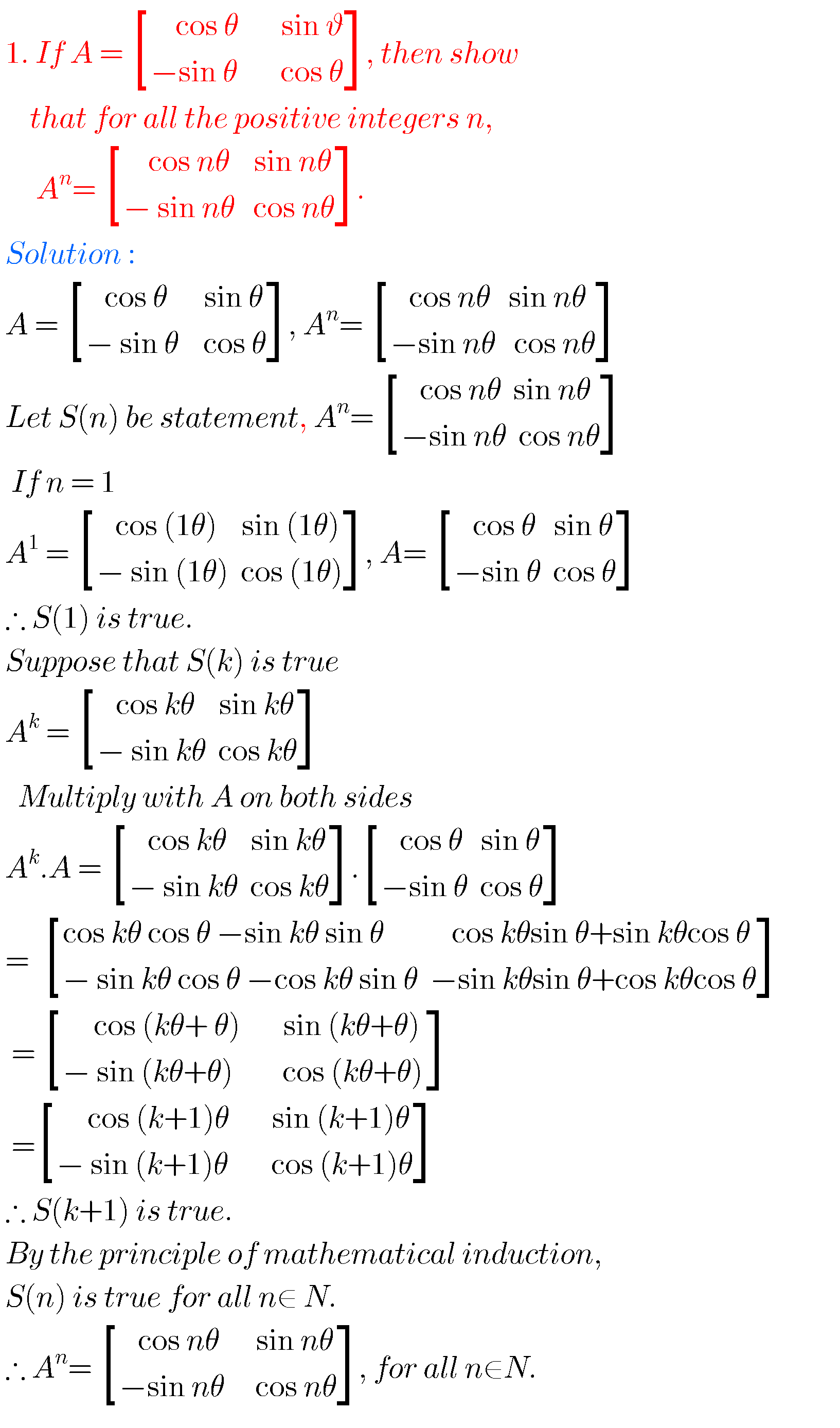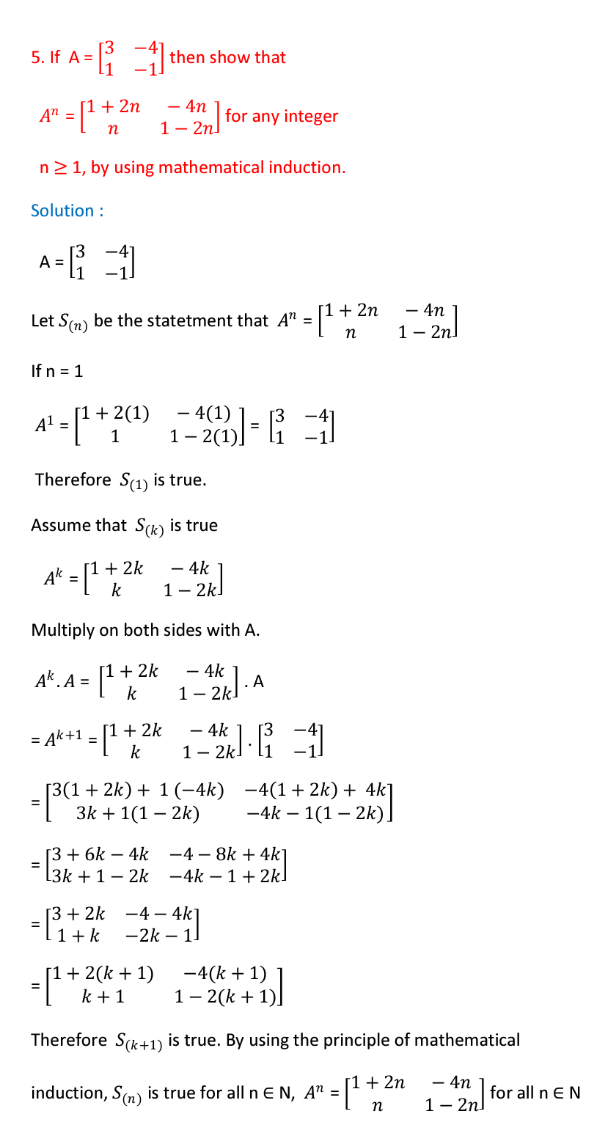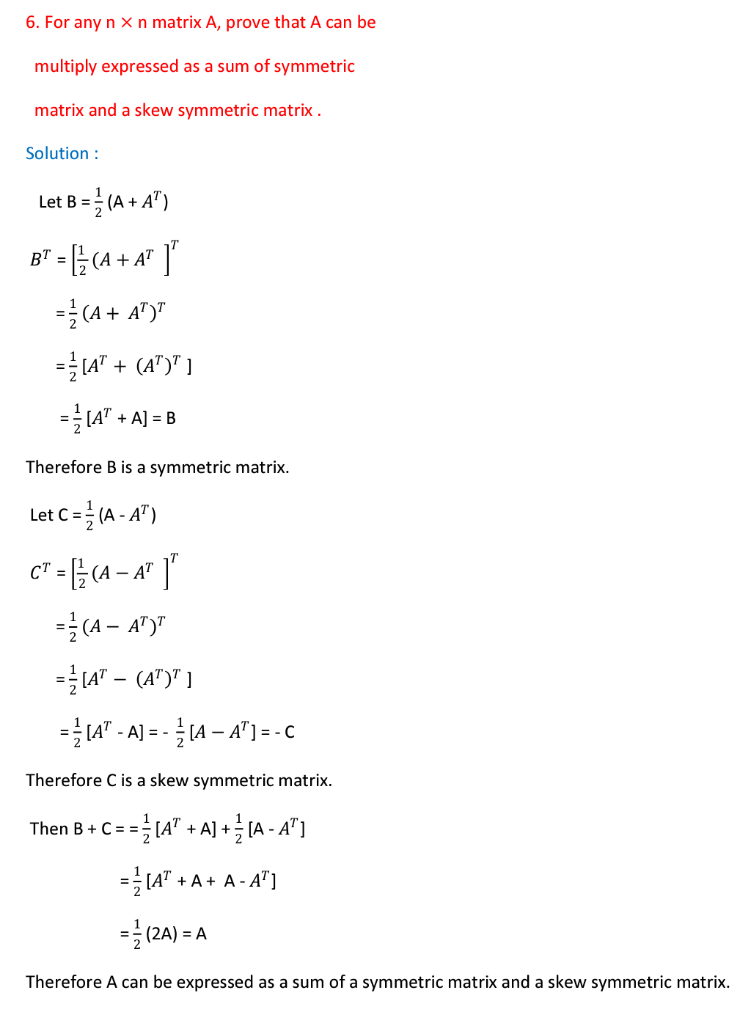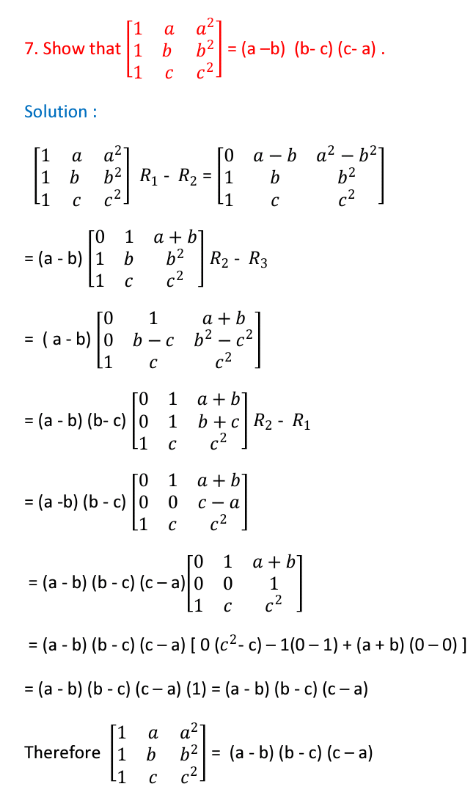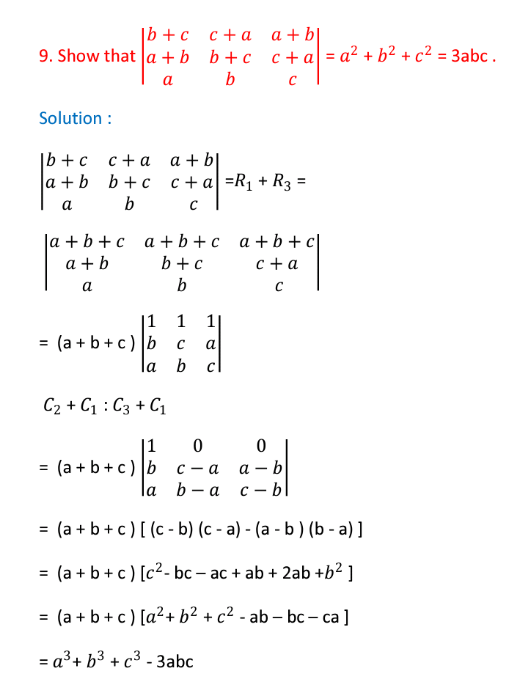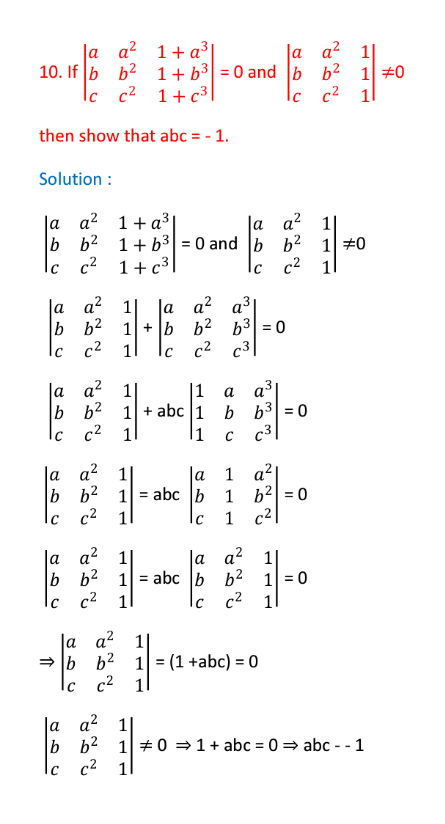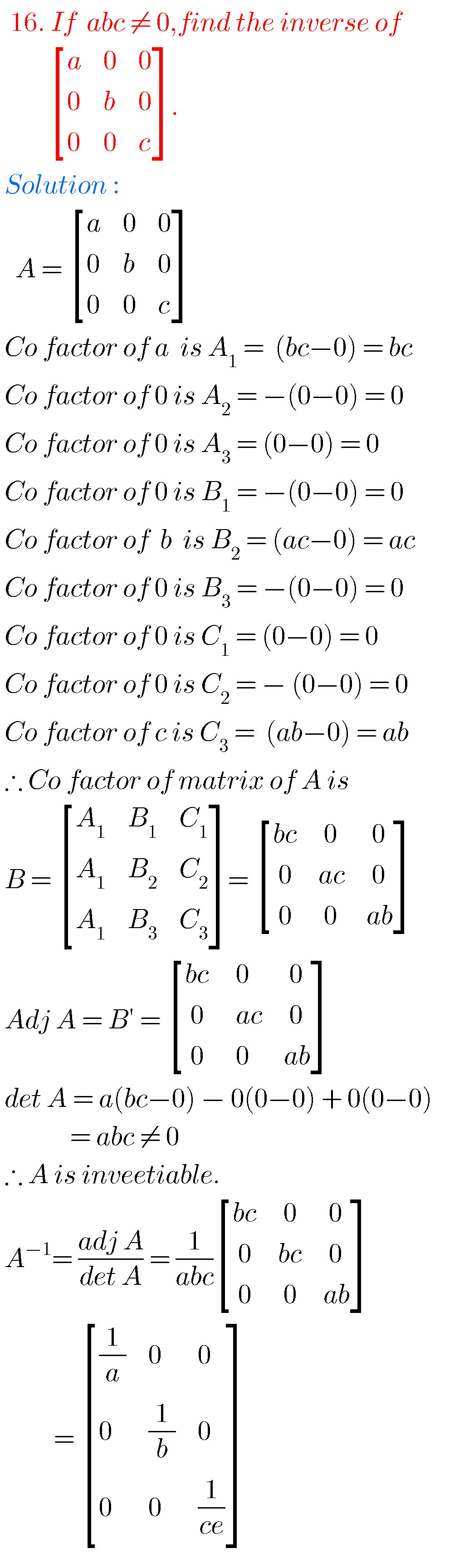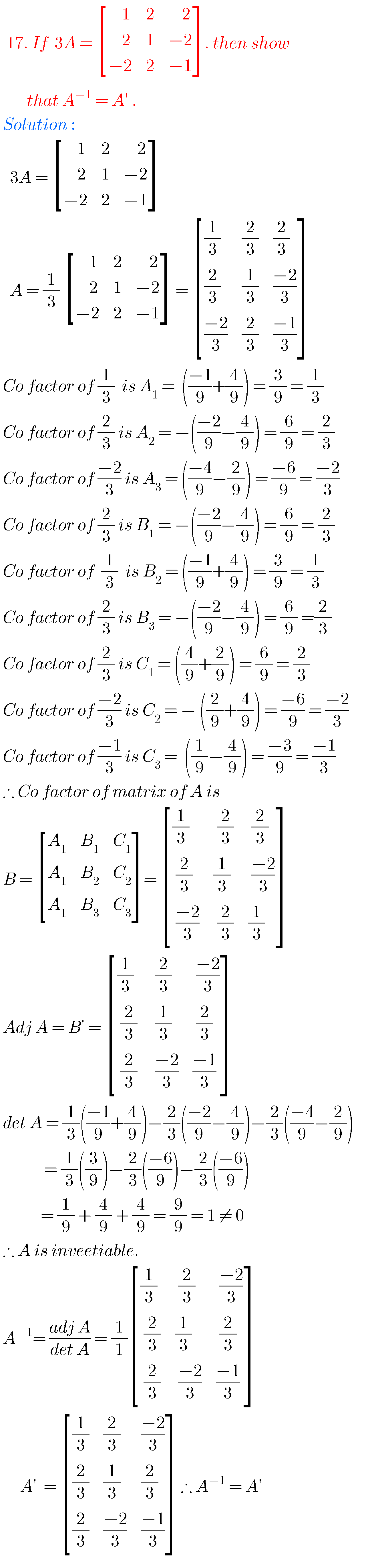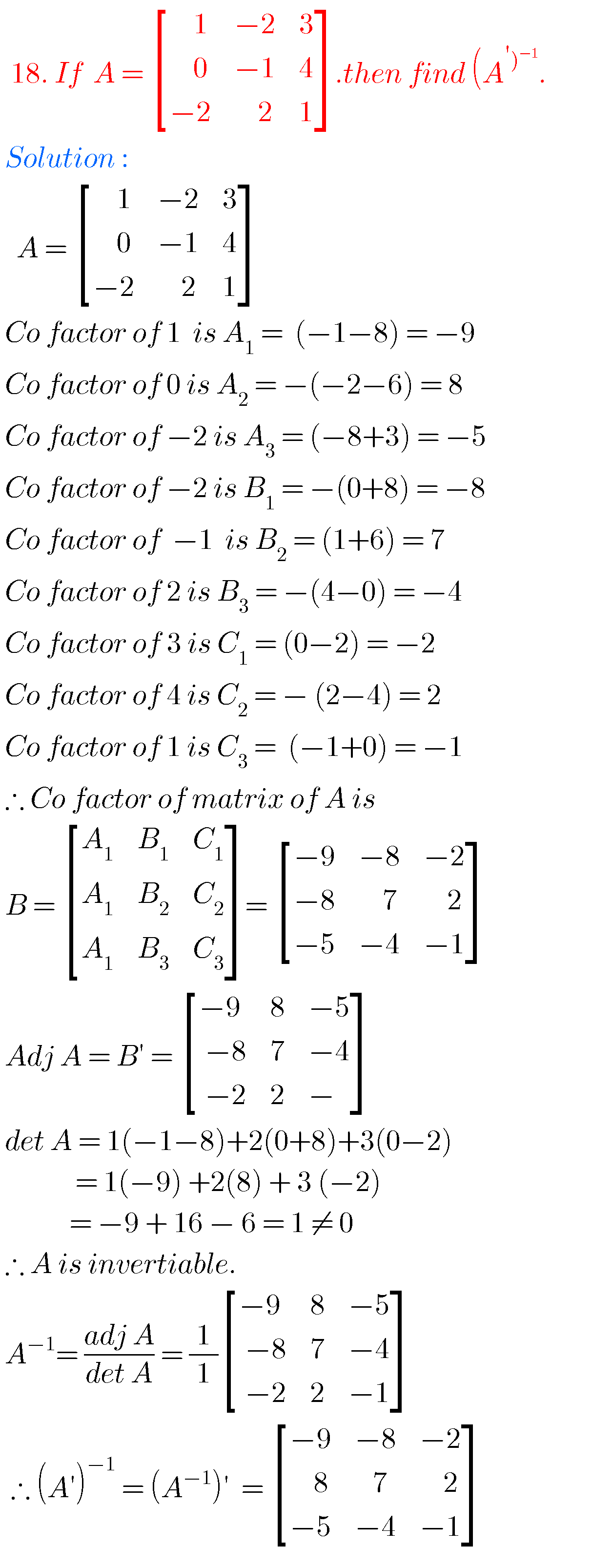### Matrix intermediate solutions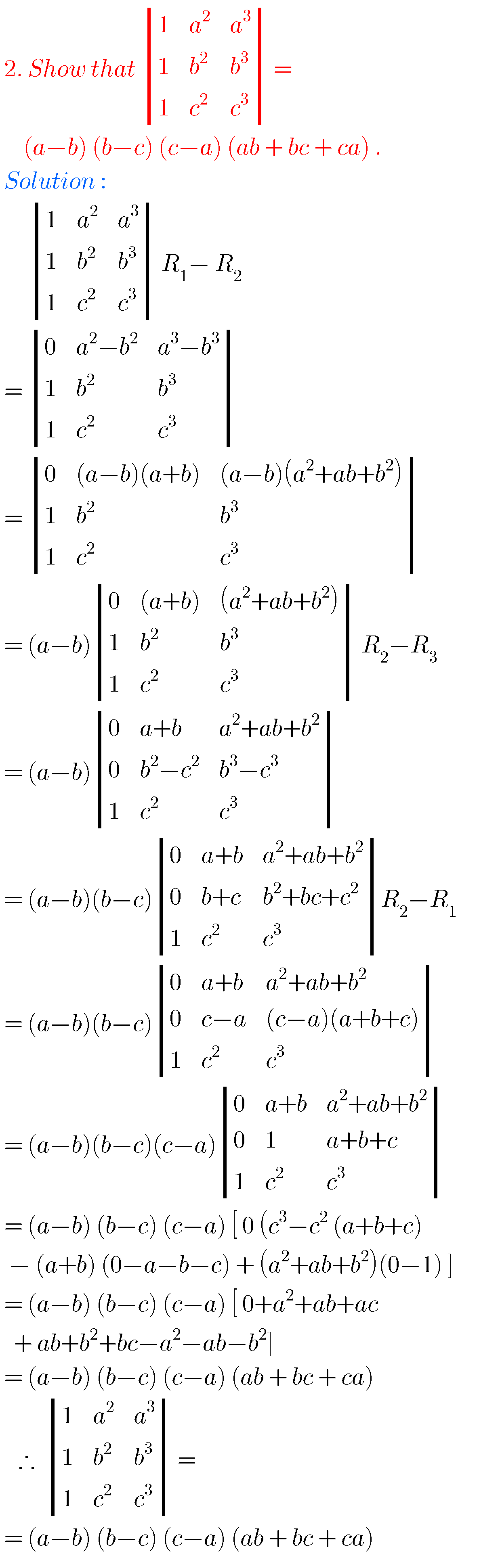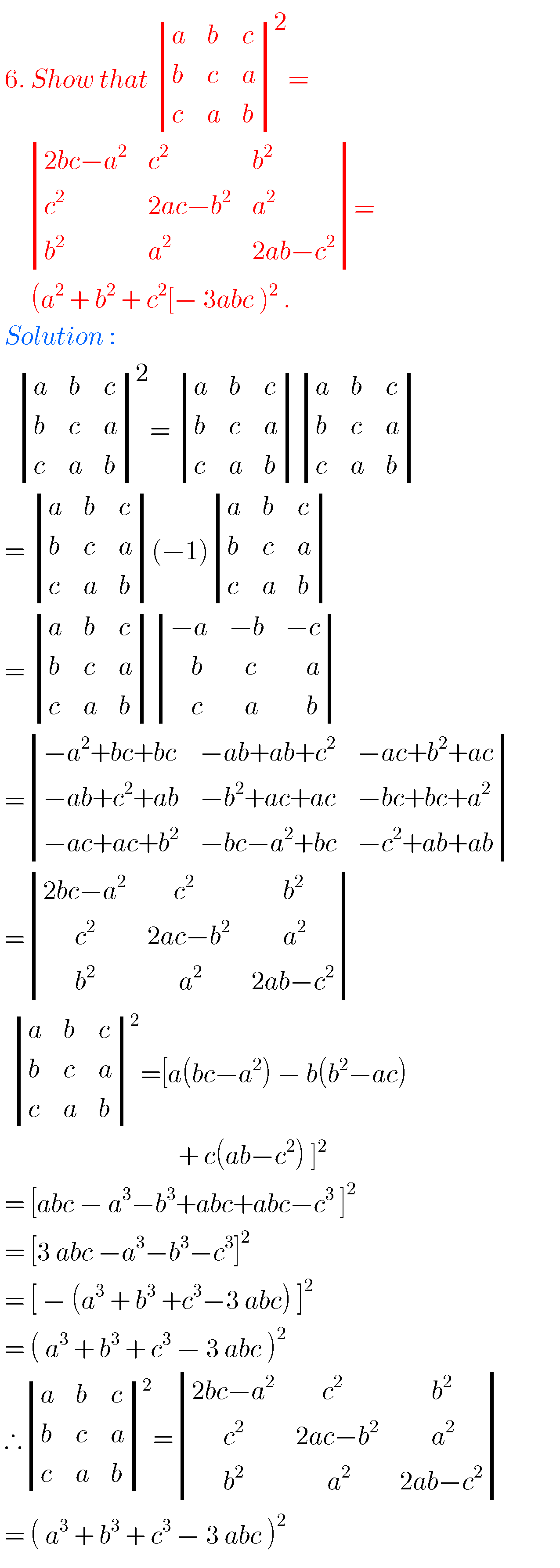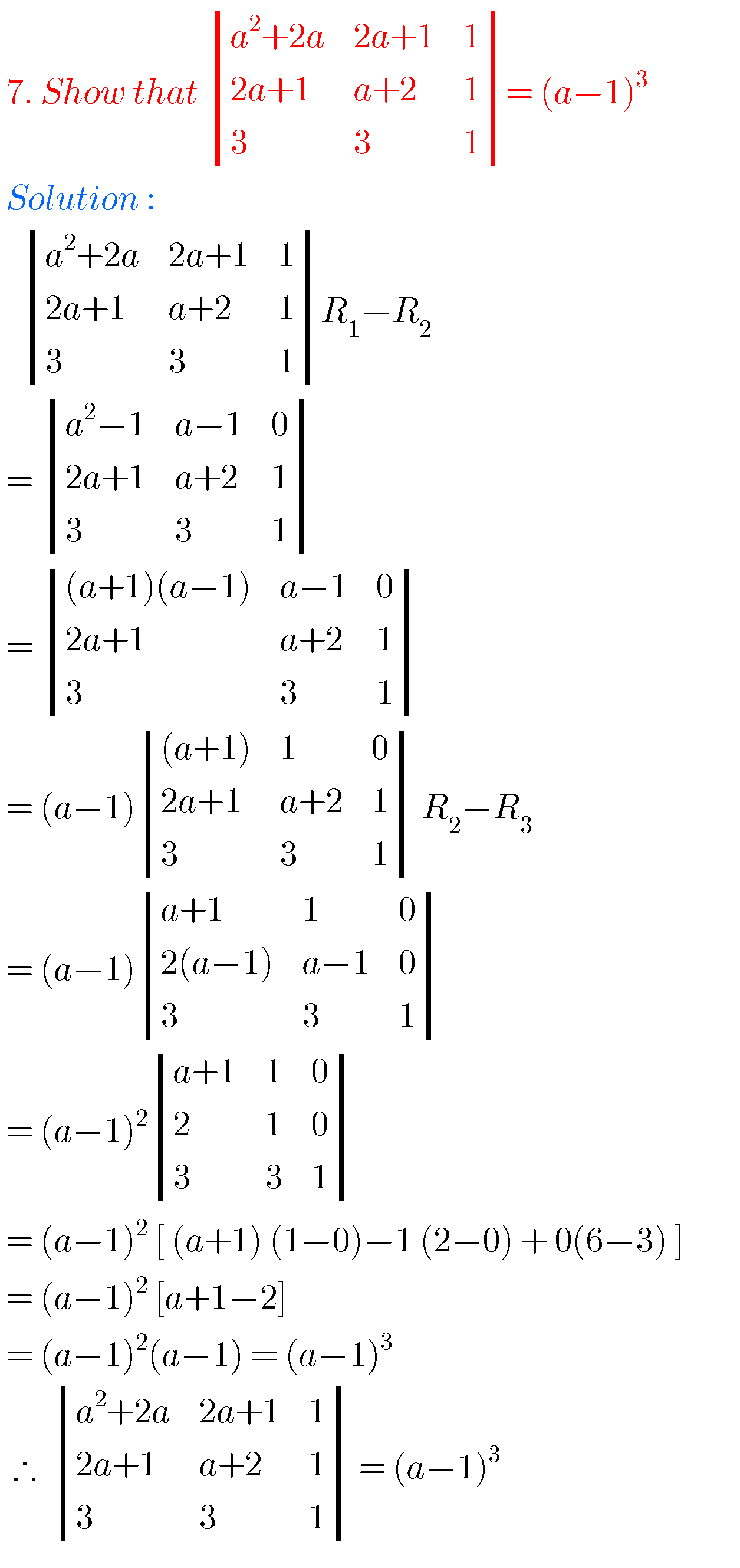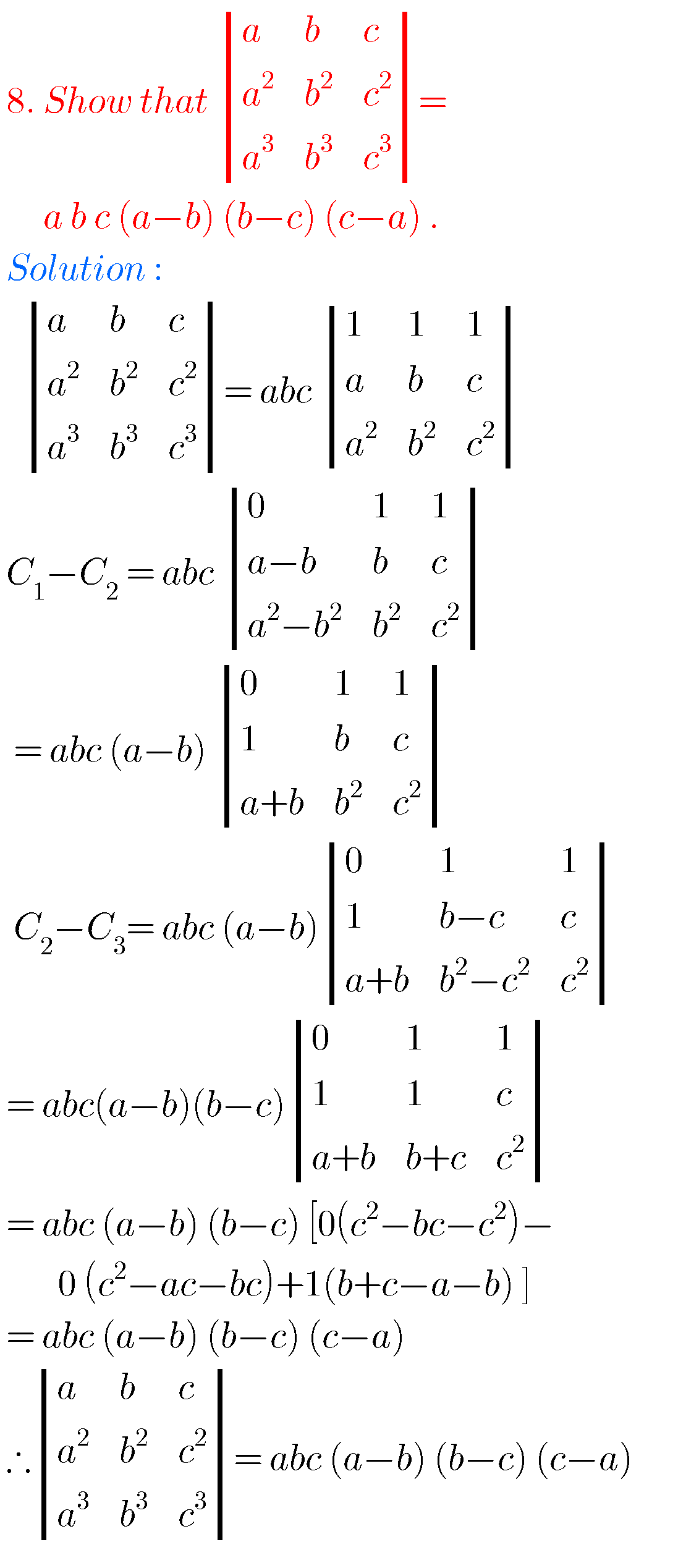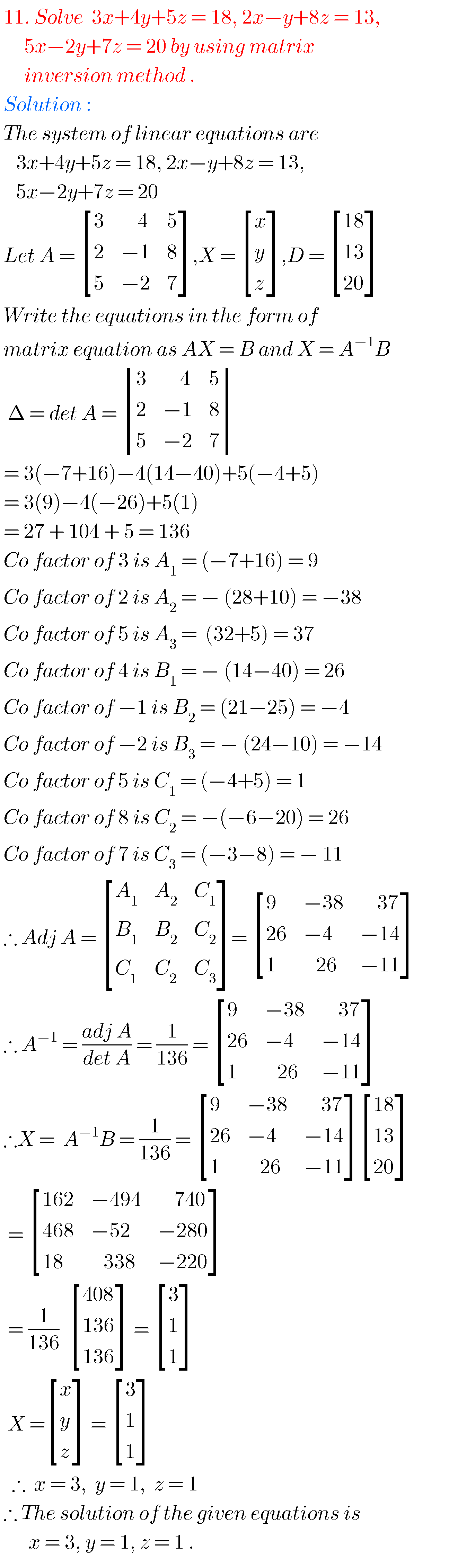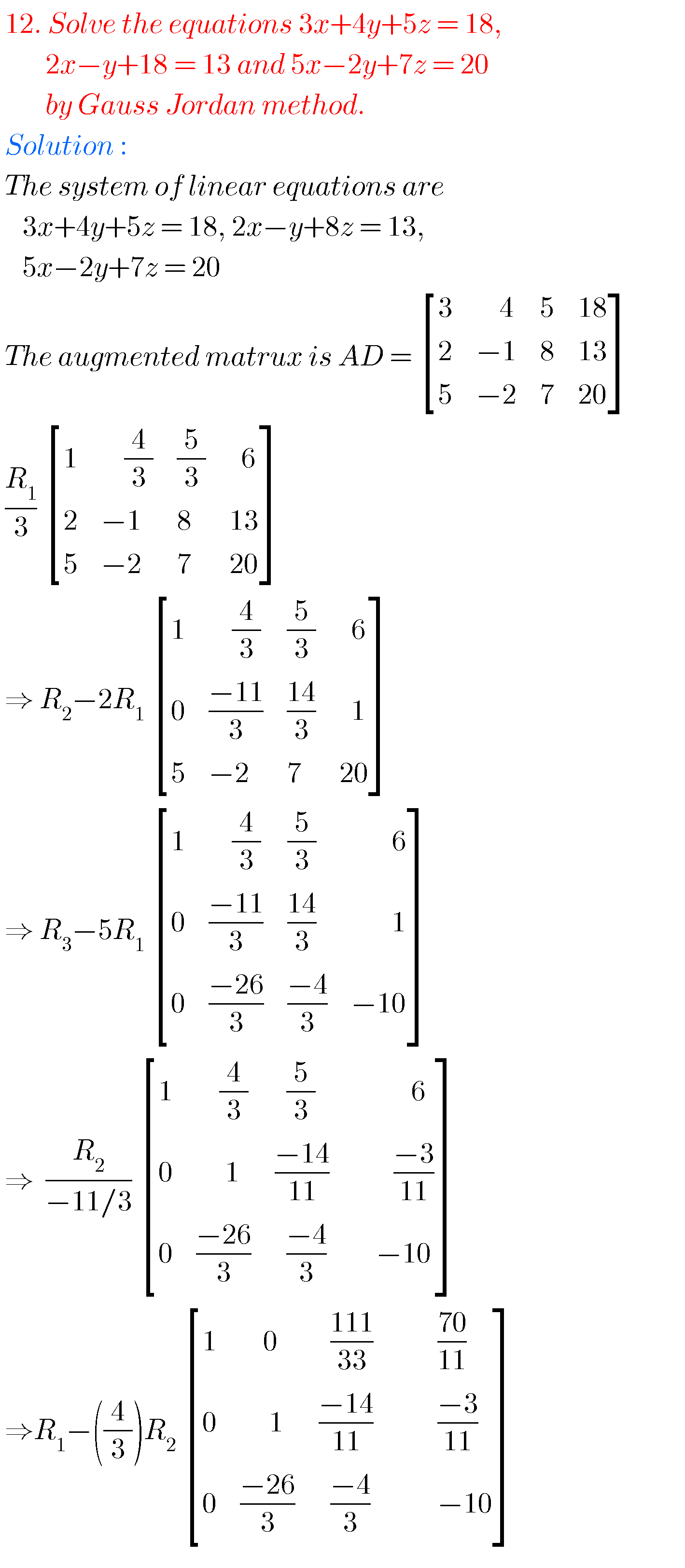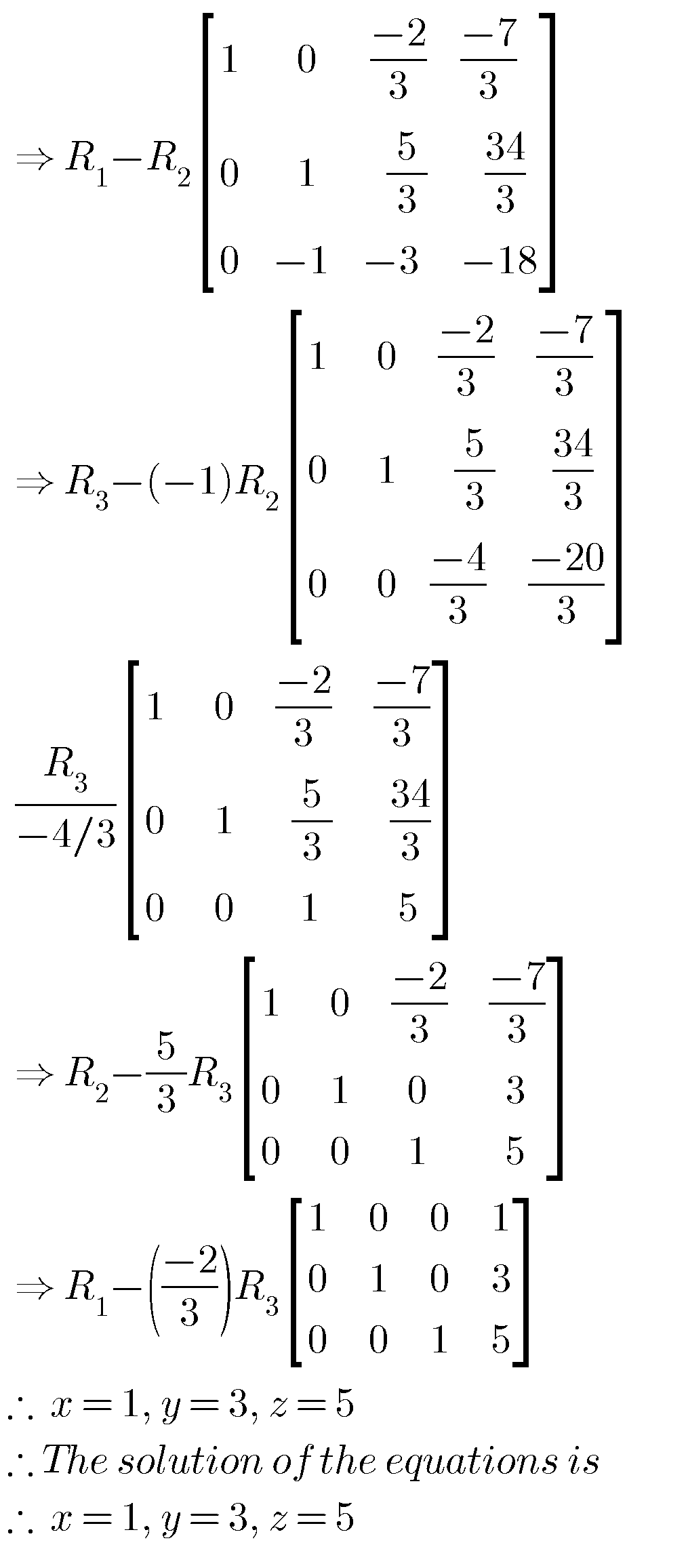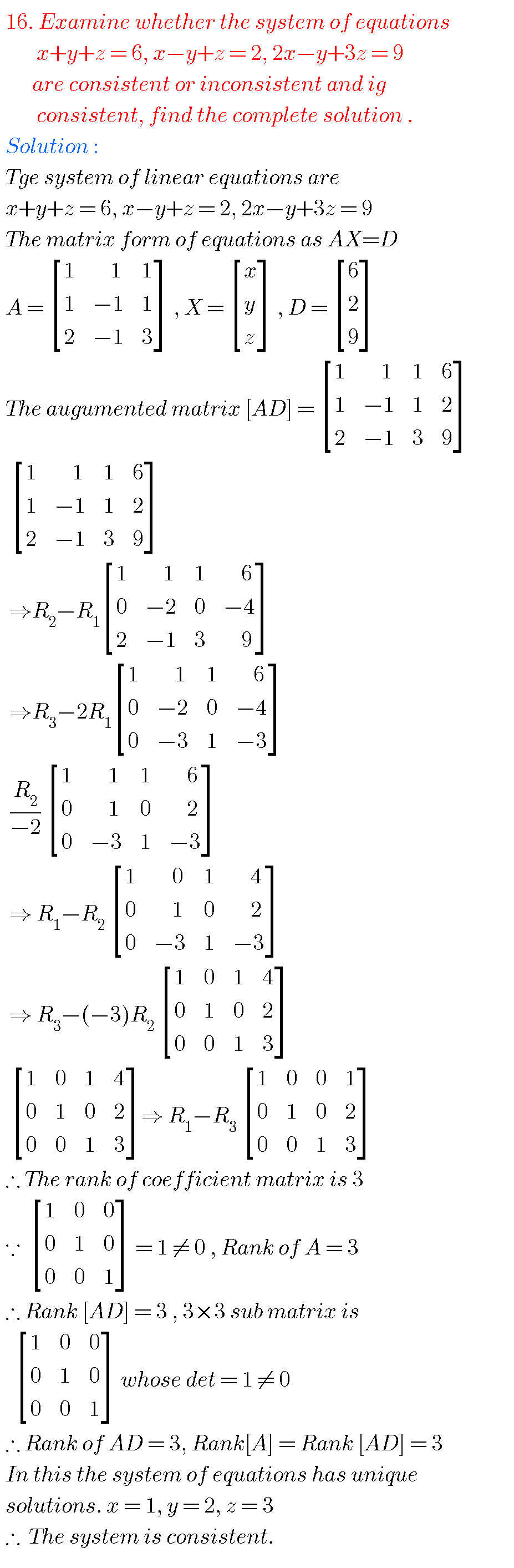Note : Observe the solutions and try them in your own methods.

Some more solutionsYou can also see the solutions for junior inter maths 1A for examination purpose.

Maths real numbers solutions class 10

Ncert maths class 6 chapter 14 solutions

Nios maths 311 book 1 Sets 1.1 solutions

### 7 thoughts on “Matrices solutions,Inter Maths 1a chapter 3 solutions”

1.1.Hi

2.I have some douts about the solutions

3.4.5.1.